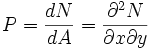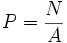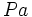# Difference between revisions of "Pressure"

## Definition

Pressure between two surfaces in contact is the normal force per unit area between them. More precisely, the pressure at a point on the surface of contact is the differential of the normal force with respect to area at the point.

In symbols:$P = \frac{dN}{dA} = \frac{\partial^2 N}{\partial x \partial y}$

Equivalently, the pressure at a point on the surface of contact is the limit of the force per unit area of smaller and smaller regions in the surface of contact containing the point.

When the normal force is uniformly distributed over the surface of contact, the pressure is given by the formula:$P = \frac{N}{A}$

Pressure is treated as a scalar, i.e., it is not given a direction, even though normal force is treated as a vector. The vector analogue of pressure is stress.

## Units and dimensions

MLT dimensions$ML^{-1}T^{-2}$: MLT;1;-1;-2
SI units$Pa$ (Pascal) or$N/m^2$ (Newton per meter squared)
Other units$Atm$ (atmospheric pressure)